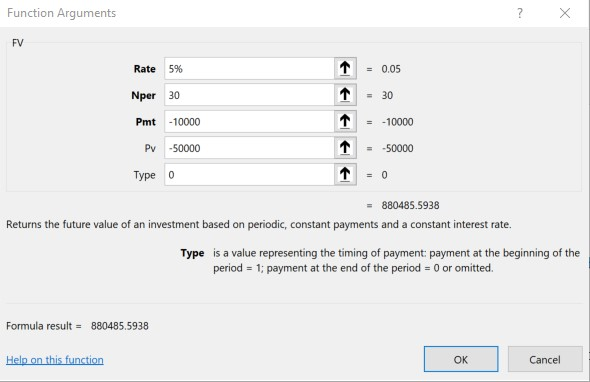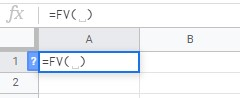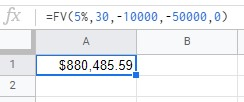By Dr. Jim Dahle, WCI Founder

Albert Einstein was reported to have said that compound interest is “the most powerful force in the universe”. Whether that is an urban legend or not, compound interest is an important financial concept for the investor to understand. If you plan to retire you want your money to grow.

Estimate how your money will grow by calculating the future value of an investment after years of compounding will help you to make goals and measure your progress toward them. Fortunately, calculating compound interest is as easy as opening up Excel or Google Sheets and using a simple function—the Future Value Formula.

## How to Calculate Compound Interest Using the Future Value (FV) Formula

### Excel

1. Open Excel.
2. Click on the Formulas tab, then the Financial tab.
3. Go down the list to FV and click on it.

A box will pop up with five values you'll need to fill in.Here's what those five boxes mean:

#### RATE

This is the interest rate or rate of return. Usually, you can just put in an annual rate of return, such as 5%. If you want to do things on a monthly basis, enter in 5%/12.

#### NPER

This is the number of periods such as years or months. If you used an annual rate above, put in the number of years, such as 30. If a monthly rate, put in the number of months in the period.

#### PMT

This is the amount you'll put into the investment on a regular basis each year. Any amount going into the investment should be a negative number, and any amount coming out of the investment should be positive. For example, if you're going to invest \$10,000 a year, enter in -10000.

#### PV

This is the present value of your investment or, in other words, the amount you already have in the investment. If, for example, you already have \$50,000 in an investment you'll enter -50000 as the PV.

#### TYPE

This is the type of payment. If you're putting the money in at the beginning of the year, put in 1. If at the end of the year, put 0 (the default option).

So, to calculate the future value of an investment that starts with a \$50,000 balance, and \$10,000 is added to it at the end of each year for 30 years, and it earns 5% a year, you will end up with \$880,485. It will look like this:Calculating Future Value in Google Sheets is a little different.

## How to Calculate Future Value in Google Sheets

1. Open Sheets.
2. Click on Insert, then Function, then Financial.
3. Select FV from the list and click on it. The spreadsheet will now look like this:Sheets does not give a prompt to fill in RATE, NPER, PMT, PV or TYPE so you'll need to enter the future value formula in yourself like this:### Playing with Future Value Variables in Excel

Once you've figured out how to calculate with the FV formula, you can play with the variables a little. Say you earn 7% a year instead of 5%. How much more do you get? You'd have \$1.33 Million, or about \$445,000 more. What if you put in \$15,000 a year instead of \$10,000 a year? That would earn you \$332,000 more. What if you invested that \$10,000 at the beginning of each year instead of the end? That would get you about \$33,000 more. What if you invested for 35 years instead of just 30? \$299,000 more.

Isn't that fun? You'll quickly realize that just a few more years of compounding or just a little higher return can make a huge difference. You also learn that in the beginning it matters much more how much you are adding to the investment (your savings rate) and in later years your rate of return matters much more. You can also see how important it is to get started as soon as possible, to maximize the years of compounding.

What do you think? Have you found the future value formula to be helpful? Why or why not? Comment below!